## Indicators

•Cited by SciELO
•Access statistics

•Cited by Google
•Similars in SciELO
•Similars in Google

## Print version ISSN 0120-5609

### Ing. Investig. vol.35 no.2 Bogotá May/Aug. 2015

#### http://dx.doi.org/10.15446/ing.investig.v35n2.50339

An algorithm for identifying the best current friend in a social network

Un algoritmo para determinar el mejor amigo actual en una red social

F. J. Moreno1, and S. Hernández2

1 Francisco Javier Moreno: Systems Engineer, Universidad de Antioquia. Ph.D Systems Engineering, Universidad Nacional de Colombia. Affiliation: Associate Porfessor Universidad Nacional de Colombia, Sede Medellín. E-mail: fjmoreno@unal.edu.co.
2 Santiago Hernández: Systems Engineer and Mathematician, Universidad Nacional de Colombia, Sede Medellín. E-mail: sahernandezt@unal.edu.co

How to cite: Moreno, F. J., & Hernández, S. (2015). An algorithm for identifying the best current friend in a social Network. Ingeniería e Investigación, 35(2), 80-88. DOI: http://dx.doi.org/10.15446/ing.investig.v35n2.50339.

ABSTRACT

A research field in the area of social networks (SNs) is the identification of some types of users and groups. To facilitate this process, a SN is usually represented by a graph. The centrality measures, which identify the most important vertices in a graph according to some criterion, are usual tools to analyze a graph. One of these measures is the PageRank (a measure originally designed to classify web pages). Informally, in the context of a SN, the PageRank of a user i represents the probability that another user of the SN is seeing the page of i after a considerable time of navigation in the SN. In this paper, we define a new type of user in a SN: the best current friend. The idea is to identify, among the friends of a user i, who is the friend k that would generate the highest decrease in the Page-Rank of i if k stops being his/her friend. This may be useful to identify the users/customers whose friendship/relationship should be a priority to keep. We provide formal definitions, algorithms and some experiments for this subject. Our experiments showed that the best current friend of a user is not necessarily the one who has the highest PageRank in the SN nor the one who has more friends.

Keywords: Social networks, friends, centrality measures, pagerank, graphs.

RESUMEN

Un campo de investigación en el área de las redes sociales (RSs) es la identificación de ciertos tipos de usuarios y de grupos. Para facilitar este proceso, una RS se suele representar mediante un grafo. Las medidas de centralidad, las cuales identifican los nodos más importantes en un grafo según algún criterio, suelen ser usadas para analizar un grafo. Una de estas medidas es el PageRank (una medida inicialmente concebida para clasificar las páginas web). Informalmente, en el contexto de las RSs, el PageRank de un usuario i representa la probabilidad de que otro usuario de la RS esté viendo la página de i luego de un tiempo considerable de navegación por la RS. En este artículo, se define un tipo de usuario en una RS: el mejor amigo actual. La idea es identificar, entre los amigos de i, quién es el amigo k que generaría el mayor decremento en el PageRank de i, si k dejara de ser amigo de i. Esto puede ser útil para identificar los usuarios/clientes cuya amistad/relación es prioritario conservar. En este artículo se presentan las definiciones formales, algoritmos y experimentos al respecto. Los experimentos demostraron que el mejor amigo actual de un usuario no es necesariamente aquel que tiene el mayor PageRank en la RS ni aquel que tiene más amigos.

Palabras clave: Redes sociales, amigos, medidas de centralidad, pagerank, grafos.

Received: April 30th 2014 Accepted: June 23th 2015

Introduction

Based on the relationships established by the members of a community, e.g., the users of a social network (SN), different types of users and user groups can be identified. For instance, with regard to users, leaders (Pedroche, 2010; Pedroche, 2012); best potential friends of a user (Moreno, Valencia, González, 2013); friends that show a distrust behavior (Ortega, 2012); and the efficient information spreaders (Kitsak et al., 2010), among others, can be identified. With regard to groups, in (Pedroche, 2010) user groups that compete for visibility in a community are identified, and in (Masuda, Kurahashi, Onari, 2012) user groups with depressive and suicidal tendencies are analyzed.

To facilitate the identification and analysis of these types of users and user groups, the community of users and their relationships are usually represented by some mechanism. For example, a SN is usually represented by a graph. Usual tools to analyze a graph are the centrality measures (Masuda, Kurahashi, Onari, 2012), which identify the most important vertices in a graph. These include the degree centrality which measures the number of links of a node, the closeness centrality determined by the length of the shortest paths from one node to the rest of the nodes of the network, the betweenness centrality that is based on the total number of shortest paths that exist among all the pairs of nodes that pass through a node, and the PageRank. The PageRank is a measure originally designed to classify web pages (Page, Brin, Motwani, Winograd, 1999). Informally, the PageRank of a web page p represents the probability that a web surfer is visiting p after a long time of navigation in the web.

In this paper, we define a new type of user in a SN based on the PageRank: the best current friend (BCF). Informally, our goal is to identify among the friends of a user i, who is the friend k that would generate the highest decrease in the PageRank of i if k stops being his/her friend. This may be useful to identify the users whose relationship (whether it be business, family or friends related) should be a priority to keep. These users are a key element for executives and for a company to get future customers. For instance, it is important for the sales executives to detect this type of users in order to keep and strengthen their relationships, e.g., to build customer loyalty, e.g., offering them extra benefits and customized services.

On the other hand, due to the changing relationships in a community (additions and deletions of both users and relationships), especially in a SN, the identification of the BCF (and other types of users) is a process that must be run whenever the number of changes in the users and in the relationships exceed a threshold established by the administrator of the SN. Indeed, the BCF of a node may change over time.

Due to the volume of users in SNs such as Facebook and Twitter (1.19 billion (Facebook Inc., 2013) and 237 million (Frier, & Spears, 2013) as of September 30, 2013 respectively), the calculation of the BCF for each node in these SNs may become a expensive computational process. For example, in our experimental environment, for a SN of 769 nodes, the calculation to generate the base matrix to identify the BCF took around two hours (see more details in the experiments section). Therefore, for SNs involving a large number of users (such as Facebook and Twitter), techniques such as sampling, pre-calculated and estimated data, parallel computing, among others must be used (Leskovec, Rajaraman, & Ullman, 2011; Bahmani, Chowdhury, & Goel, 2010; Lee, 2003).

The present paper is organized as follows: in Section 2, we present the basic elements of the PageRank algorithm; in Section 3, we formally introduce the concept of the BCF based on the PageRank; in Section 4, we present and analyse some experiments; in Section 5, we review some related works; and in Section 6, we conclude the paper and outline future work.

Basic definitions

The users and their relationships in a SN may be represented by a graph. For example, consider a SN with n = 5 users represented by a directed graph GSN = (N, E), where N represents the set of nodes {1, 2, 3, 4, 5} and E the set of edges {(1, 2), (1, 3), (2, 1), (2, 3), (2, 4), (3, 1), (3, 4), (4, 2), (4, 5),(5, 2)}, see Figure 1. An edge (i, j) indicates that i is friend of j (i points to j). Note that this representation supports both unidirectional and bidirectional relationships, e.g., in Twitter a user w follows a user z but z not necessarily follows w, i.e., they show a unidirectional relationship.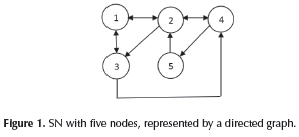Our goal is to classify the nodes (users) of a SN applying the PageRank method (Pedroche, 2010a; Pedroche, 2012; Page, Brin, Motwani, & Winograd, 1999). Note that in a SN it is reasonable to assume that each user points to at least one friend (i.e., an outlink). This is a mandatory condition to apply the PageRank method to SNs analysis, i.e., there must not be dangling nodes (Pedroche, 2010a).

To apply the PageRank method, first we build a connectivity matrix H = (hij) ∈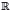nxn, 1 ≤ i, j n, that represents the links of each node. If there exists a link from node i to node j, i j, then hij = 1, otherwise hij = 0; if i = j then hii = 0, see Figure 2.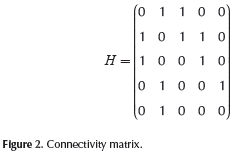From H matrix we build the row stochastic matrix P = (pij) ∈nxn, 1 ≤ i, j n. A matrix is row stochastic if the sum of the elements of each of its rows is 1. P is calculated by dividing each element hij by the sum of the elements of row i of H, see Figure 3. Note that we assume that there do not exist dangling nodes then this sum (in each row) cannot be zero.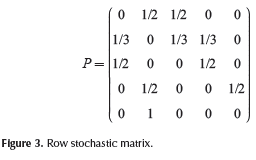The PageRank method requires that the P matrix, in addition to being row stochastic, must be primitive. A non-negative square matrix is primitive (Varga, 2009) if the number of distinct eigenvalues of the matrix whose absolute value is equal to the spectral radius p(P) is 1, where p(P) is the maximum value (in absolute value) of its eigenvalues. In order to ensure this property (and still preserving the row stochastic property), we apply the following transformation (Page, Brin, Motwani, & Winograd, 1999; Pedroche, 2007): G = aP + (1 - a)evT, where G is known as Google matrix; a is a damping factor, 0 < α < 1, and represents the probability with which the surfer of the network moves among the links of the H matrix; and (1- α) represents the probability of the surfer to randomly navigate to a link which is not among the links of H. Note that if a = 1, then G = P, i.e., we would be working with the original P matrix. Usually, a is set to 0.85, a value that was established by Brin and Page, the creators of the PageRank method (Page, Brin, Motwani, & Winograd, 1999; Pedroche, 2007). In (Becchetti,& Castillo, 2006; Boldi, Santini, & Vigna, 2005; Boldi, 2005) the effect of several values of a is analyzed.

On the other hand, e ∈nx1 is the vector of all ones, and vTe = 1. v is called personalization or teletransportation vector and may be used to affect (to benefit or to harm) the ranking of the nodes of the network (Pedroche, 2007): v = (vi) ∈nx1: vi > 0, 1 ≤ i n. Usually, v = (1/n), and is known as the basic personalization vector. However, if we want to affect the ranking of a specific node i, v may be defined as follows: Let 0 < ε < 1 then vi = (vij)nx1: vij = ε/(n - 1) for i j, vii = 1 -ε,. Thus, when e is close to zero, the ranking of node i tends to increase, but if ε is close to one, its ranking tends to decrease. A commonly used value in specialized literature for ε is 0.3 (Pedroche, 2010a).

Note that the constraint vTe = 1 allows us to define a v vector such that benefits (or harms) the ranking of several nodes simultaneously. For example, if v = (7/20 7/20 1/10 1/10 1/10) then the ranking of nodes 1 and 2 tend to be benefited whereas the ranking of nodes 3, 4, and 5 tend to be harmed. We denote PPR (Personalized PageRank) as the PageRank of a node using some pre-scribed personalization vector vi and we denote PRi the PageRank vector computed using vi.

In Figure 4, we show the G matrix which was computed with a = 0.85 and the basic personalization vector.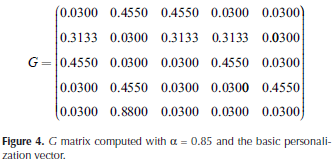From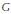matrix we can compute the PageRank vector π To compute vector π we consider the following system of equations πT=πT,where πT= [q1 q2 q3 q4q5]. In addition, to ensure that n is a probability vector, we also consider the equation: q1 + q2 + q3 + q4 + q5 = 1. For the running example, the system of equations is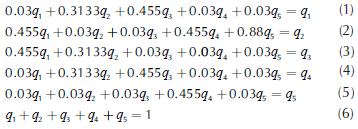We solved the system using MATLAB; results are showed in Table 1. The results show that node 2 has the highest PageRank whereas node 5 has the lowest one.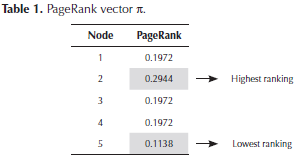As a second example, we compute vector π with the personalization vector of node 3, i.e., PR3with ε= 0.3, i.e., v3= (0.08 0.08 0.7 0.08 0.08). The corresponding G matrix is showed in Figure 5.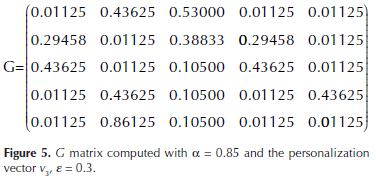The system of equations is: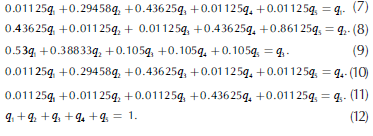The resulting PR3 vector is showed in Table 2.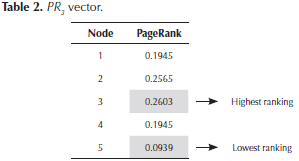Note that node 3 improved its ranking with regard to the PageRank vector n of Table 1 (it changed from 0.1972 to 0.2603).

The BCF

We introduce the concept of the BCF of a node in a SN. The BCF of a node i is the node k of the SN, k≠i, H[k, i] = 1, such that if k stops being friend of i (k stops pointing to i), k is the node that generates the highest decrease in the PageRank of i. That is, let GSN = (N, E) be the original graph that represents the SN. Let Πi(GSN) denote the i component of the PPR for some personalization vector v. Given i ∈ N, let: Q(i ) = { j ∈ N: i j, (j, i) ∈ Ε }, i.e., the set of nodes that point to i. Let Ε'(j, i) = Ε - {(j, i)}, with some j ∈ Q(i ), i.e., the original set of edges Ε minus the edge from j to i, and let GSN'(j, i) = (N, Ε'(j, i)). Then we say that k ∈ Q(i) is the BCF of i if the following condition holds: Π(GSN'(k,i)) = min(Πi(GSN'(j,i))), j ∈ Q(i).

We define the Current Friend PageRank Vector of a node i as follows: CFPRVi=Πit (GSN'(j, i)), 1 ≤j≤ n, j ≠ i. Note that if Π[j, i] = 0 (i.e., j is not friend of i) or if j cannot be disconnected (because in doing so, the sum of the elements of each row of H would be zero) then CFPRVi(j) = N/A (not applicable).

The next algorithm computes the CFPRVi. Let CFPRVi(k), 1 ≤ k n, k i, be the minimum value in CFPRVi, then k is the BCF of i.

Algorithm CFPRV(i, H:n x n).

Input: i: The node for which the CFPRV will be computed

H: Connectivity matrix

Preconditions: H is a matrix of order n, n > 1∧1≤ in ∧(H[p,q] = 0 v H[p,q] = 1 ∀ p,q, 1 ≤ p,q ≤ n) ∧(∀ p,1 ≤ p ≤ n, Σnq=1 H[p, q] ≠ 0)

Output: CFPRV: Current Friend PageRank Vector of node i.

Postconditions: CFPRV is a vector of dimension n, (n > 1) ∧ (∀ p, 1 ≤ p ≤ n, 0 ≤ CFPRV[p] ≤ 1∧ CFPRV[p] = N/A).

1. j = 1; //Variable to iterate through the nodes of the SN

2. While j ≤ n Do

3. IF (H[j,i] = 1 AND numberofOutlinks(j) >1) THEN /*numberofOutlinks() computes the number of outlinks of a node. If numberofΟutlinks(j) ≤ 1 then j cannot be disconnected because the sum of the elements of each row of H cannot be zero*/

4. auxH = H; //auxH is a copy of H matrix

5. auxH[j,i]= 0; // j stops being friend of i

6. Compute PageRank vector using auxH matrix

7. CFPRV[j] = PageRank[i] /*Get PageRank of node i and store it in CFPRV*/

8. ELSE CFPRV[j] = N/A; /*j does not point to i or cannot be disconnected from it*/

9. END IF

10. j = j + 1;

11. END WHILE

12. RETURN CFPRV

Next, we prove the correctness of our algorithm.

Loop invariant

For the j-th iteration (1 ≤ jn), the dimension of CFPRV is j,

and ∀ k, 1 ≤ k j, CFPRV[k] stores the PageRank of node i, when disconnecting node k (if possible) or N/A otherwise:

(CFPRV: (j- 1) x 1) ∧ (j ≤ n) ∧ (∀k, 1 ≤ k≤ j, CFPRV[k] = PageRank(auxH, i))

Initialization

For j = 1, from the preconditions we know that n> 1, then j < n. Moreover, CFPRV has dimension 0.

Maintenance

For the j-th iteration (1 < j < n), the dimension of CFPRV is (j - 1) and j < n. Considering the truth value of (H [j,i] = 1 AND number of Οutlinks(j) > 1) there are two cases:

• If true then CFPRV[j] = PageRank[i], then CFPRV: jx 1.

• If false then CFPRV[j] = N/A.

In any case j = j + 1, then (CFPRV: j x 1) ∧ (j + 1 ≤ n).

Termination

In the last iteration (n-th), CFPRV has dimension (n - 1) and j = n. Again, considering the truth value of (H[j, i] = 1 AND number of Outlinks(j) > 1) there are two cases:

• If true then CFPRV[j] = PageRank[i], then CFPRV: j x 1.

• If false then CFPRV[j] = N/A.

In any case j = j + 1, then (CFPRV: jx 1) ∧ (j + 1 > n) and because of the second condition of the invariant the loop ends.

Example. Consider the SN of Figure 1. Currently, the PageRank of node 2 (the node with the highest PageRank) is 0.2944. In Table 3, we show the change in the PageRank of this node depending on the node that has been disconnected (CFPRV2). In this example, node 1 is the BCF of node 2 because if it is disconnected, it will be the node that decreases the most the PageRank of node 2. Note that node 2 currently has another connected node, node 5. However, if this node is disconnected, the PageRank method becomes inapplicable because node 5 will be left without any outlinks.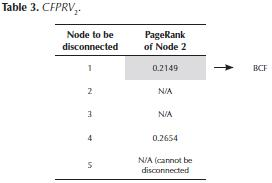Using our CFPRV algorithm, we can create the Current Friend PageRank Matrix (CFPRM), i.e., we compute the CFPRV for each user of the SN, as we show in the following algorithm:

Algorithm CFPRM(H: n x n).

Input: H: Connectivity matrix.

Preconditions: H is a matrix of order n, n >1 (H[p, q] = 0 v H[p, q] = 1 ∀ p, q, 1 ≤ p, q ≤ n)∧( ∀ p,1 ≤ p ≤ n, Σnq-1 H[p, q] ≠ 0)

Output: CFPRM = Current Friend PageRank Matrix

Postconditions: CFPRM is a matrix of order n, ∧ (∀p, q 1 ≤ p, q ≤ n, 0 ≤ CFPRV[p, q] ≤ 1 a CFPRV[p,q] = N/A).

1. For i = 1 to n

2. CFPRM [i] = CFPRV(i, H)

3. End for

4. Return CFPRM

Since the length of this article is limited, we do not present a complete correctness proof of this algorithm. The main part is the loop invariant: For the j-th iteration (1 ≤j ≤ n), the order of CFPRM is n x( j - 1), and ∀ k, 1≤ k j, CFPRM[k] is the result of computing CFPRV(k, H): (CFPRM n x (j- 1)) ∧ (j ≤ n) ∧ (V k, 1 ≤ k≤j, CFPRM[k] = CFPRV(k, H)).

In Table 4, we show the CFPRM matrix for the SN of Figure 1. For example, the BCF of the node 4 is the node 3.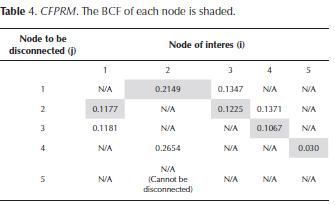Experiments

The algorithms were implemented in MATLAB. The experiments were carried out for a real subnetwork of 769 nodes of Facebook, Caltech-2005 (Traud, Kelsic, Mucha, & Porter, 2011). The calculation of the CFPRM matrix was executed in a laptop with an Intel Core I7 processor with 4 GB of RAM memory and the calculation took almost two hours.

Experiment 1 : the analyses were focused on two groups of nodes: the 10 with the highest and the lowest PageRank in the SN. To determine the effect of removing the BCF from a node, the node with higher PageRank in the SN was initially chosen, the node identified with number 623. In Figure 6 we show the effect on the PageRank of the node 623 when removing its BCF (the node identified with number 104), and the following nine nodes that decrease the most its PageRank.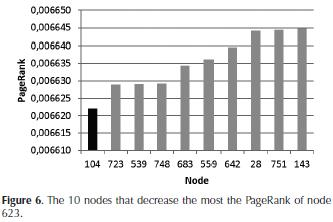Results: note that if the node 623 loses its BCF (node 104), its PageRank falls from 0.006683691154 to 0.0066220198284 (e.g., a difference of 0.000061671), whereas if it loses the tenth node (node 143) that most decreases its PageRank, its PageRank falls to 0.006644868 (i.e., a difference of 0.0000388). While these differences are in the order of 1E-05, they may be significant (Leskovec, Rajaraman, & Ull-man, 2011). In fact, such a decrease in the PageRank could cause that a node ceases to be the "leader" of the SN (i.e., it will no longer be the node with the highest PageRank in the SN). In Figure 7, the 10 nodes with the highest PageRank in the SN are shown, indicating their PageRank when they lose their respective BCF.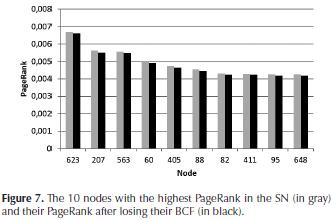In our SN, the node with the highest PageRank (node 623) has a significant advantage regarding the PageRank of the following nodes with the highest PageRank in the SN. For example, the difference with the second node with higher PageRank (node 207) is 0.00104413; for this reason, the decrease that node 623 suffers when losing its BCF is not enough to lose its position as the leader of the SN.

However, in other nodes, the loss of their BCF may change their ranking (according to their PageRank) in the SN. For instance, consider node 82, which is currently in the seventh position in the SN according to its PageRank. If it looses its BCF, its Page Rank will be lower than the one of node 411 (which is currently in the eighth position in the SN).

Experiment 2: In a second experiment, we compared the PageRank of the 10 nodes that decrease the most the PageRank of node 623 with the PageRank of the 10 nodes that decrease the least its PageRank. The results are shown in Figure 8.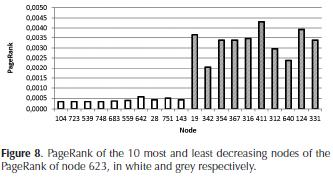Results: It is interesting to note that the nodes that decrease the most the PageRank of node 623 have a very low PageRank compared to the ones that decrease the least their PageRank.

Experiment 3: next, we found the BCF for each node of the SN, and we determined which nodes appeared more frequently as the BCF of other nodes. In addition, the PageRank of these nodes and their ranking in the SN according to their PageRank were calculated. The results are shown in Table 5.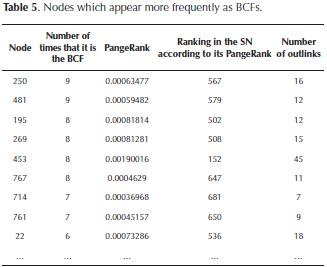Results: note that the nodes which appear more frequently as BCFs of other nodes have few outlinks and their PageRank is low in comparison to other nodes in the SN. For instance, the nodes identified with numbers 250 and 481 are the BCFs of nine nodes in the SN; and according to their PageRank, these two nodes occupy the positions 567 and 579 in the SN, respectively. This suggests, at least in this SN, that the nodes that appear more frequently as BCFs tend to occupy lower positions in the SN (according to their PageRank), but they are significant for the PageRank of other nodes.

A possible explanation for this behavior is shown in Figure 9. As the PageRank represents the probability to reach a node j after a long time t of navigation in the SN, and if k is a node which is connected to j, then the more outlinks k has, the less will be the probability to reach each of them. Therefore, any path containing k and ending in j will have a lower probability as the number of outlinks of k increases due to the rule of the product for probabilities. Informally, this means that the probability to reach a node j from k will be higher if k has fewer outlinks.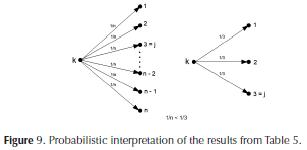Experiment 4: In our last experiment, we compared our proposed method (based on PageRank) to find the BCF of a node and the following alternative (based on degree centrality). Our goal was to analyze how the PageRank of a node is affected when losing its BCF or its node (friend) with the greatest number of outlinks. To this end, we selected the 10 nodes of the SN with the highest PageRank. For each of these nodes i, we found the node j (connected to i), which had the greatest number of outlinks. In s 6 we also show the PageRank of each node i if it lost the friendship of j (j stops being friend of i), the PageRank of each node i if it lost its BCF, and the difference between those two values.

Results: as expected, the results evidenced that from the point of view of the PageRank, the loss of a friend with many outlinks is less "severe" than the loss of the BCF. Note again that although the differences (last column of Table 6) are in the order of 1E-05, these could mean the loss of positions of a node in the SN according to their PageRank.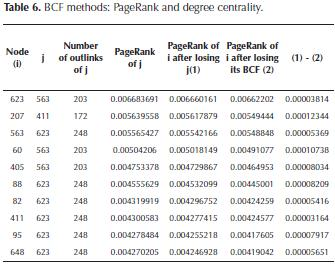Related work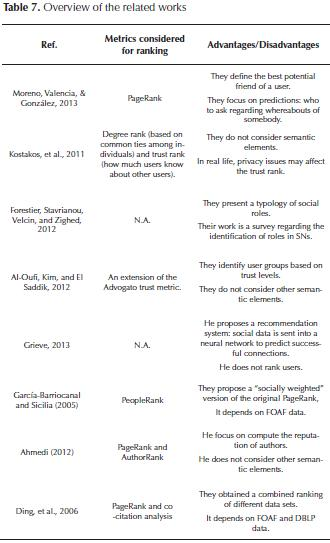Conclusions and future work

In this paper, a new type of user of a SN, the BCF, was formally defined. Based on the PageRank, the most important friend of a user i was determined. This friendship is the most important one to keep since in case it gets lost, this would seriously affect the PageRank of i. The identification of the BCF could be decisive for the user when keeping the visibility and influence in the SN. In addition, we presented a corresponding algorithm to identify it as well as its correctness. Although experiments with SNs with a larger number of users and with other SNs such as Twitter (it was tested with a Facebook subnetwork of 769 users) are required, the results evidenced, e.g., that the BCF of a node is not necessarily the one, among all the friends, that has the highest PageRank or the one who has more friends.

As future work, we consider the following: to define the BCF in terms of other measures (e.g., those mentioned in the related work section), to compare the results among them, and to determine correlations if there is any. For instance, if a node k is the BCF of a node i when considering a measure c then, how close is k to be the BCF of i if another measure were considered? Another future work could be the development of a visual tool that allows the analysts to identify, in a friendly way, the BCF of each node, and that also allows them the interactive manipulation of the SN (addition and deletion of nodes/relationships), and that shows the way the BCF of each node is affected given these changes. This could contribute to the understanding of how the relationships of other users of the SN affect a node i and to its corresponding BCF. At the same time, this could lead to the identification of "the best external friendship" with regard to a node i, i.e., among all the couples of friends in a SN (couples that do not include i), which is the couple that generate the highest decrease in the PageRank of i if this couple fell out. A third possible future work is to rank users with regard to their behavior or sentiments (Liu, 2015). For example, we could define the BCF of a user i based on sentiments, i.e, who is the friend k that would generate the highest decrease in the level of happiness of i if k stops being his/her friend.

References

Ahmedi, L., Abazi-Bexheti, L., & Kadriu, A. (2011). A Uniform Semantic Web Framework for Co-authorship Networks. IEEE 9th International Conference on Dependable, Autonomic and Secure Computing (pp. 958-965). Sydney, Australia. DOI: 10.1109/dasc.201 1.159.         [ Links ]

Ahmedi, L. (2012). AuthorRank + FOAF: ranking for co-authorship networks on the web. IEEE/ACM International Conference on Advances in Social Networks Analysis and Mining (pp. 315-321). Istanbul, Turkey. DOI: 10.1109/asonam.2012.60.         [ Links ]

Al-Oufi, S., Kim, H. N., & El Saddik, A. (2012). A group trust metric for identifying people of trust in online social networks. Expert Systems with Applications, 39(18), 13173-13181. DOI: 10.1016/j.eswa.2012.05.084.         [ Links ]

Bahmani, B., Chowdhury, A., & Goel, A. (2010). Fast incremental and personalized PageRank. VLDB Endowment, 4(3), 173-184. DOI: 10.14778/1929861.1929864.         [ Links ]

Ball, B., & Newman, M. E. J. (2013). Friendship networks and social status. Network Science, 1(01), 16-30. DOI: 10.1017/nws.2012.4.         [ Links ]

Becchetti, L., & Castillo, C. (2006). The distribution of Page-Rank follows a power-law only for particular values of the damping factor. 15th international conference on World Wide Web (pp. 941-942). Edinburgh, UK. DOI: 10.1 145/1135777.1135955.         [ Links ]

Boldi, P., Santini, M., & Vigna, S. (2005). PageRank as a function of the damping factor. 14th international conference on World Wide Web (pp. 557-566). Chiba, Japan. DOI: 10.1145/1060745.1060827.         [ Links ]

Boldi, P. (2005). TotalRank: ranking without damping. Special interest tracks and posters of the 14th international conference on World Wide Web (pp. 898-899). Chiba, Japan. DOI: 10.1145/1062745.1062787.         [ Links ]

Ding, Y., Scharffe, F., Harth, A., & Hogan, A. (2006). AuthorRank: Ranking Improvement for the Web. International Conference on Semantic Web and Web Services Conference (pp. 1-7). Las Vegas, USA.         [ Links ]

Forestier, M., Stavrianou, A., Velcin, J., & Zighed, D. A. (2012). Roles in social networks: Methodologies and research issues. Web Intelligence and Agent Systems: An international Journal, 10(1), 117-133.         [ Links ]

García Barriocanal, E., & Sicilia, M. A. (2005). Filtering information with imprecise social criteria: A FOAF-based backlink model. 4th conference of the European Society for Fuzzy Logic and Technology (pp. 1094-1098). Barcelona, Spain.         [ Links ]

Golbeck, J., & Rothstein, M. (2008). Linking Social Networks on the Web with FOAF: A Semantic Web Case Study. 23th AAAI Conference on Artificial Intelligence (pp. 1138-1143). Chicago, USA.         [ Links ]

Grieve, A. (2013). Predicting likelihood of a successful connection between unconnected users within a social network using a learning network. U.S. Patent No. 8595167 (B1). Mountain View, CA: U.S. Patent and Trademark Office.         [ Links ]

Jeong, Y. K., Song, M., & Ding, Y. (2014). Content-based author co-citation analysis. Journal of Informetrics, 8(1), 197-211. DOI: 10.1016/j.joi.2013.12.001.         [ Links ]

Kitsak, M., Gallos, L.K., Havlin, S., Liljeros, F., Muchnik, L., Stanley, H. E., & Makse, H. A. (2010). Identification of influential spreaders in complex networks. Nature Physics, 6(11), 888-893. DOI: 10.1038/nphys1746.         [ Links ]

Kostakos, V., Venkatanathan, J., Reynold, B., Sadeh, N., Toch, E. , Shaikh, S. A. & Jones, S. (2011). Who's your best friend? Targeted privacy attacks in location-sharing social networks. ACM Conference on Ubiquitous Computing (pp. 177-186). Beijing, China.         [ Links ]

Lee, C. P. (2003). A fast two-stage algorithm for computing PageRank and its extensions. [Technical report], 1-9.         [ Links ]

Leskovec, J., Rajaraman, A., & Ullman, J.D. (2011). Mining of Massive Datasets. New York, USA: Cambridge University Press.         [ Links ]

Liu, B. (2015). Sentiment Analysis: Mining Opinions, Sentiments, and Emotions. Cambridge, UK: Cambridge University Press. DOI: 10.1017/CBO9781139084789.         [ Links ]

Liu, X., Bollen, J., Nelson, M. L., & Van de Sompel, H. (2005). Coauthorship networks in the digital library research community. Information Processing and Management, 41(6),1462-1480. DOI: 10.1016/j.ipm.2005.03.012.         [ Links ]

Masuda, N., Kurahashi, I., & Onari, H. (2013). Suicide Ideation of Individuals in Online Social Networks. PLoS ONE,8(4), e62262.         [ Links ]

Moreno, F., Valencia, A., & González, A. (2013). My best potential friend in a social network. In Tu, X.M., White, A.M. and Lu, N. (Ed), Social Networking: Recent Trends, Emerging Issues and Future Outlook, 113-124.         [ Links ]

Nascimento, M. A., Sander, J., & Pound, J. (2003). Analysis of SIGMOD's co-authorship graph. SIGMOD Record, 32(3),8-10. DOI: 10.1145/945721.945722.         [ Links ]

Ortega, F.J., Troyano, J.A., Cruz, F.L., Vallejo, C.G., & Enríquez, F. (2012). Propagation of trust and distrust for the detection of trolls in a social network. Computer Networks, 56 (12), 2884-2895. DOI: 10.1016/j.comnet.2012.05.002.         [ Links ]

Page, L., Brin, S., Motwani, R., & Winograd, T. (11, Nov, 1999). The PageRank citation ranking: bringing order to the web. Retrieved from: http://ilpubs.stanford.edu:8090/422/.         [ Links ]

Pedroche, F. (2010a). Ranking nodes in social network sites using biased PageRank. 2° Encuentro de Álgebra Lineal Análisis Matricial y Aplicaciones, ALAMA-2010. Universidad Politécnica de Valencia (pp. 1-7). Valencia, España.         [ Links ]

Pedroche, F. (2010b). Competitivity Groups on Social Network Sites. Mathematical and Computer Modelling, 52(7-8), 1052-1057. DOI: 10.1016/j.mcm.2010.02.031.         [ Links ]

Pedroche, F. (2012). A model to classify users of social networks based on PageRank. International Journal of Bifurcation and Chaos, 22 (7), 1-14. DOI: 10.1142/s0218127412501623.         [ Links ]

Pedroche, F. (2007). Métodos de cálculo del vector PageRank. SeMA Boletín de la Sociedad Española de Matemática Aplicada, 9(39), 7-30.         [ Links ]

Traud, A.L., Kelsic, E.D., Mucha, P.J., & Porter, M.A. (2011). Comparing community structure to characteristics in online collegiate social networks. SIAM Review, 53(3), 526-543. DOI: 10.1137/080734315.         [ Links ]

Varga, R. S. (2009). Matrix iterative analysis. Berlin: Springer.         [ Links ]